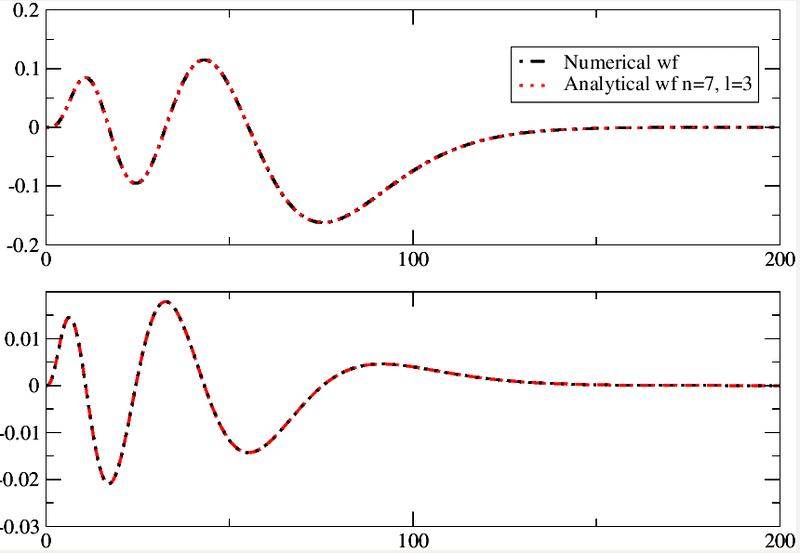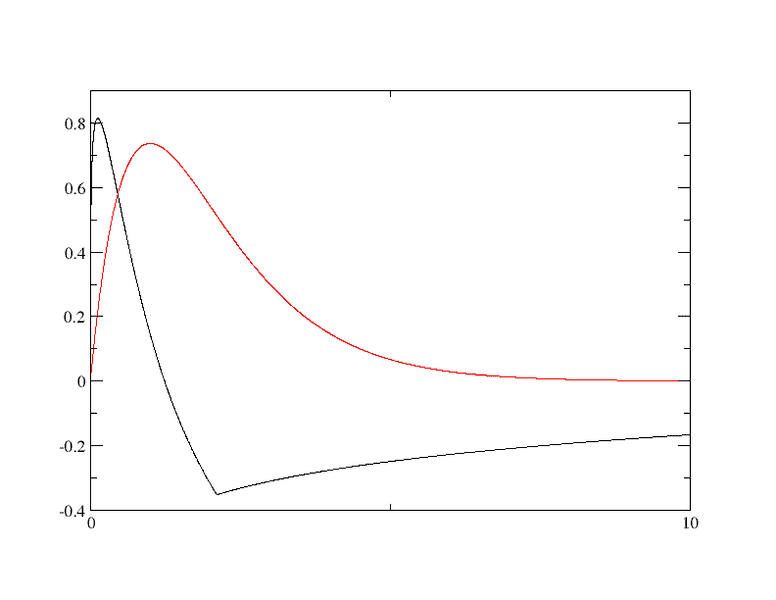# Numerov method on a exponential grid mesh

• Telemachus

#### Telemachus

Hi there. I was trying to implement Numerov method for solving second order differential equations (you can see some information of Numerov algorithm at here: https://en.wikipedia.org/wiki/Numerov's_method ). At the beginning I've used a uniform grid mesh, all points equally spaced. I'm working in Fortran, and the program I wrote worked fine on that mesh, I've tried different things and contrasted what the method gave with the analytical solutions, everything went just great. But then I wanted to use a different kind of grid mesh, so I've implemented an exponential grid mesh. When I've started to run the program on this exponential grid mesh, with points spaced like

$$x_i=x_0(e^{t_i}-1)$$ and $$t_i=(i-1)h$$

the solution started to look like compressed at the origin, where points are more dense in the gridmesh. So I came to think that probably this was because of Numerov method being a multi step method derived on a uniform grid mesh, that the problem was maybe due that the algorithm doesn't have in account how the points in the domain are spaced when I use a grid mesh which is not uniform, so when I've took a domain with discrete points given by an exponential function, adjacent points were not equally spaced anymore, and that changed the metric somehow. Is this appreciation correct? I have never done this before, so I'm just "trying". I haven't read any book on how to implement this sort of things. If you have any reference on where to look for the implementation of variable grid mesh that would be great.

So then what I tryied to do was to modify the algorithm to work in this exponential domain. So I rederived a formula by using the chain rule in the taylor expansion. But then the method doesn't look that nice anymore, I have many derivatives of all orders including a first order one, which I can't extract from the differential equation as it is done in the standard Numerov method.

$$y''(x)=-g(x)y(x)+s(x)$$

Well, any idea, correction or comment will be welcome. Thank you in advance.

Note to the moderation: I've originally posted this issue in here: https://www.physicsforums.com/threads/variable-grid-mesh-in-numerovs-method-fortran.870657/ but then I thought that this subforum was more appropriate. Please, don't be mad at me for posting this again in here, just delete the other topic if necessary.

The first thing as a computer guy to ask you about without even understanding the details of what you're doing, is: have you looked at potential numeric issues with it? These come up all the time with exponential stuff. The computer uses a floating point type to represent the number. So if you sum a large float and a small float, the small float just disappears, because of the rounding, so you lose information and get garbage out of complex computations. I don't know much about FORTRAN, but a quick way to test if you think this may be it is to try changing from single precision to double precision floats, and see if it gets a little better. I don't know if this is your issue, but its a simple thing to rule out.

•Telemachus and jim mcnamara
Thank you very much for your response first of all.

I've been working with double precision all the time. Actually, the result doesn't look like garbage, but instead it seems to be this kind of "metric" problem. Of course, this is what I've deduced, and I'm not totally sure this is the problem. Probably someone working in numerical analysis could say if that could be the problem (I have never before worked with variable grid meshes, and I've been "inventing" what I did, so I'm not much sure of anything at all). But, in principle, this is what I thought. The Numerov method uses information of previous and forward steps, and when I've changed the grid mesh to the exponential, the thing actually looks like if it where compressing the wave function in the region where the x points are more dense. I'll post a picture to clarify. Numerov method uses a Taylor expansion of the wavefunction, now my domain has real numbers that grows exponentially, not linearly (as in the uniform grid). I thought that this non uniformity could introduce some sort of different 'metric' in the wave function. After all, when one approximates a derivative by finite differences, is using this linearity, and this 'metric' to approximate the differentials. But I'm not sure, because is the first time I'm doing this, but I thought that could be the problem. I've tried to modify Numerov method, by considering a composite function ##y(e^x)## and using the chain rule in the Taylor expansion, but then the algorithm looses all of its nice simplicity, and there appears derivatives of all orders, including a first order which didn't allow me to proceed, because I would have to integrate the function I'm looking for, and things get more complicated.

This is for example a result I've obtained previous to changing the grid (that is, with a uniform grid using Numerov method):Upwards are the numerical and analytical wavefunction (the program calculates the analytical wf by means of Laguerre polynomials). And downwards the derivatives. The program propagates from the outward to the inward region, and from the inward to the outward, and then matches both solutions, normalize, etc. I'm using the discontinuity at the matching point as a convergence criteria.

As you may see I have an excellent agreement if I use a proper number of points in the grid mesh to propagate the numerical solution.

Now, when I've tried to implement the exponential grid this is what I obtain (this is for the ground state):It looks awful, but the exterior region is actually not that bad. The thing is that when the program matches both solutions (inward and outward) it uses the value of the function propagating from the inward to the outward region at the matching point, and at that point the outward solution is already negative, so it looks like the matching procedure is inverting the wave function with respect to the x axis. What the wavefunction looks like is like if it were being compressed in the interior region, where there are a bigger number of points (the discrete grid is more dense in that region).

#### Attachments

Last edited:
Hmm, sounds like interesting stuff, but a really dense problem. I think this is the first question: is the unexpected result due to computation (such as numeric errors) a bug or coding issues (you entered the wrong thing, or aren't sure how to implement an algorithm in FORTRAN) or mathematical errors? If you can shrink the problem down to a specific field, I think it will be easier to answer.

•Telemachus
Well, I personally think that the issue is due to the discretization, basically I wanted to know if someone have worked with variable grids with this kind of multistep methods, and what would be expected to see by the trained eye. Is there anyway to save this problem or am I condemned with it? perhaps I reached as far as it can be taken, but I'm just not sure. I'm actually not sure about my hypothesis of this behaviour being given by the discretization it self, and if it is a reasonable thing trying to modify Numerovs method using the chain rule. I actually am not sure about the whole thing, but it worked pretty well on the uniform grid. I have checked many things in the program. I would like to know if this kind of problem is to be spected when changing the grid, I am no expert in numerical analysis.

Hmm. Sounds like its hard to pin down where the problem really lies, to reduce it to a pure computer science problem. I'll let it stand then, good luck!

•Telemachus
Great, thank you :D you helped me a lot in trying to clarify my problem.